• 孪生神经网络专题
2021-06-02 15:27:16

# 孪生神经网络（Siamese Network）

## 可以解决的问题？

• 孪生神经网络可解决以下两种分类问题：
• 第一类，分类数量较少，每一类的数据量较多，比如ImageNet、VOC等。这种分类问题可以使用神经网络或者SVM解决，只要事先知道了所有的类
• 第二类，分类数量较多（或者说无法确认具体数量），每一类的数据量较少，比如人脸识别、人脸验证任务。

## 何为“孪生”？

• Siamese：”连体“，”孪生“，来源于泰国（暹罗）的连体双胞胎（Siamese Twins）

• Siamese Network 具有两个结构相同，且权值共享的子网络。分别接收两个输入 X 1 X_{1} X 2 X_{2} ，将其转换为向量 G W ( X 1 ) G_{W}(X_{1}) G W ( X 2 ) G_{W}(X_{2}) ，再通过某种距离度量的方式计算两个输出向量的距离 E W E_{W}

在低维空间，任意两个样本：

• 如果它们是相同类别，空间距离尽量接近0
• 如果它们是不同类别，空间距离大于某个间隔
• 训练样本：

训练样本的形式应该表示成为一个三元组，即 t u p l e ( X 1 , X 2 , Y ) tuple (X_{1},X_{2},Y) 将其转化为向量 G W ( X 1 ) G_W(X_{1}) G W ( X 2 ) G_{W}(X_{2}) ，再通过某种距离度量的方式计算出两个输出向量的距离 E W E_{W}

• 孪生方法：

寻找一个映射函数，能够将输入图像转换到一个特征空间，每个语句对应一个特征向量，通过一些简单的“距离度量”（比如欧式距离）来表示向量之间的差异，最后通过这个距离来拟合输入图像的相似度差异（语义差异）。

## 采用什么方法解决？

• Siamese Network 思路：

孪生神经网络提出了一种思路，将输入映射成以恶特征向量，采用L1正则化指标衡量两个向量之间的距离，定量表示输入向量之间的差异。孪生神经网络每次需要输入两个样本作为一个样本对来计算损失函数，提出了用于训练的Contrastive Loss用于孪生神经网络的训练。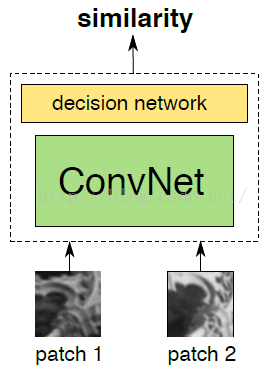## 孪生神经网络结构

Siamese Network 结构：—— 类似于差分放大器## 评价指标 之 损失函数

LOSS函数设计的基本准则应该是

L ( W ) = ∑ i = 1 P L ( W , ( Y , X 1 , X 2 ) i ) L ( W , ( Y , X 1 , X 2 ) i ) = ( 1 − Y ) L G ( E W ( X 1 , X 2 ) i ) + Y L I ( E W ( X 1 , X 2 ) i ) \begin{aligned} \mathcal{L}(W) &=\sum_{i=1}^{P} L\left(W,\left(Y, X_{1}, X_{2}\right)^{i}\right) \\ L\left(W,\left(Y, X_{1}, X_{2}\right)^{i}\right) &=(1-Y) L_{G}\left(E_{W}\left(X_{1}, X_{2}\right)^{i}\right) +Y L_{I}\left(E_{W}\left(X_{1}, X_{2}\right)^{i}\right) \end{aligned}
其中，数学符号表示

• Y Y 表示 X 1 , X 2 X_{1}, X_{2} 是否属于同一类:
• 若为同类，则为0;
• 若为不同类，则为1。
• P P 表示输入的总样本数， i i 表示当前样本的下标。
• L G L_{G} 表示两个样本为同类时的损失函数, L I L_{I} 表示两个样本未不同类时的损失函数。
• 使用Contrastive Loss的任务主要是设计合适的 L G L_{G} L I L_{I} 损失函数, 当为同类时，使得 L G L_{G} 尽可能 小; 当不同类时，使得 L I L_{I} 尽可能大。文中给出的函数如下图, 现在也不常用了，推导步骤略。

在Caffe框架下，损失函数定义为
L = 1 2 N ∑ n = 1 N y D W 2 + ( 1 − y ) max ⁡ ( margin ⁡ − D W , 0 ) 2 L=\frac{1}{2 N} \sum_{n=1}^{N} y D_{W}^{2}+(1-y) \max (\operatorname{margin}-D_{W}, 0)^{2}

其中，
D W ( X 1 , X 2 ) = ∥ X 1 − X 2 ∥ 2 = ( ∑ i = 1 P ( X 1 i − X 2 i ) 2 ) 1 2 D_{W}\left(X_{1}, X_{2}\right)=\left\|X_{1}-X_{2}\right\|_{2}=\left(\sum_{i=1}^{P}\left(X_{1}^{i}-X_{2}^{i}\right)^{2}\right)^{\frac{1}{2}}

• D W D_{W} 代表两个样本特征 X 1 X_{1} X 2 X_{2} 的欧式距离（向量差的二范数）

• P P 表示样本的特征维数

• Y Y 为两个样本是否匹配的标签

• Y = 1 Y=1 代表两个样本相似或者匹配
• Y = 0 Y=0 代表两个样本不匹配
• m a r g i n margin 为设定的阈值，也可以成为边际价值。有一个边际价值表示超出该边际价值的不同对不会造成损失。这是有道理的，因为你只希望基于实际不相似对来优化网络，但网络认为是相当相似的。

• Y = 1 Y=1 时，如果 X 1 X_{1} X 2 X_{2} 之间距离大于 m m ，则不做优化——省时省力
• Y = 0 Y=0 时，如果 X 1 X_{1} X 2 X_{2} 之间的距离小于 m m ，则调整参数使其距离增大到 m m
• N N 为样本个数

## 详谈损失函数

• 损失函数还有更多的选择

Siamese network的初衷是计算两个输入的相似度,。左右两个神经网络分别将输入转换成一个"向量"，在新的空间中，通过判断cosine距离就能得到相似度了。cosine是一个选择，exp function也是一种选择，欧式距离什么的都可以，训练的目标是让两个相似的输入距离尽可能的小，两个不同类别的输入距离尽可能的大。其他的距离度量没有太多经验，这里简单说一下cosine和exp在NLP中的区别。

• 根据实验分析，cosine更适用于词汇级别的语义相似度度量，而exp更适用于句子级别、段落级别的文本相似性度量。其中的原因可能是cosine仅仅计算两个向量的夹角，exp还能够保存两个向量的长度信息，而句子蕴含更多的信息。

### 损失函数 Python 实现

import torch
import numpy as np
import torch.nn.functional as F

class ContrastiveLoss(torch.nn.Module):
"Contrastive loss function"
def __init__(self, m=2.0):
super(ContrastiveLoss, self).__init__()
self.m = m

def forward(self, output1, output2, label):
d_w = F.pairwise_distance(output1, output2)
contrastive_loss = torch.mean((1-label) * 0.5 * torch.pow(d_w, 2) +
(label) * 0.5 * torch.pow(torch.clamp(self.m - d_w, min=0.0), 2))

return contrastive_loss


其中，F.pairwise_distance(x1, x2, p=2)

pairwise_distance(x1, x2, p) Computes the batchwise pairwise distance between vectors x 1 , x 2 x_{1},x_{2} using the p-norm.

( ∑ i = 1 n ( ∣ x 1 − x 2 ∣ p ) ) 1 p x 1 , x 2 ∈ R b × n \begin{array}{c} \left(\sum_{i=1}^{n}\left(\left|x_{1}-x_{2}\right|^{p}\right)\right)^{\frac{1}{p}} \\ x_{1}, x_{2} \in \mathbb{R}^{b \times n} \end{array}

## 小结

孪生神经网络的直接用途就是衡量两个输入之间的差异/相似程度。

孪生神经网络的用途：

• 词汇语义相似度分析
• 手写体识别
• 问题匹配
• Kaggle上Quora的Question Pair比赛，即判断两个提问是否为同一个问题

# 改进的Siamese网络 （2-channel networks）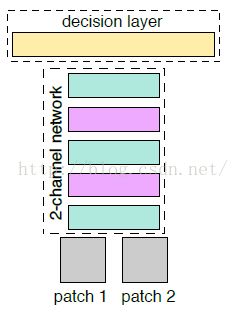## Siamese 网络(2-branches networks)的大体思路：

1. 让patch1、patch2分别经过网络，进行提取特征向量(Siamese 对于两张图片patch1、patch2的特征提取过程是相互独立的)
2. 然后在最后一层对两个两个特征向量做一个相似度损失函数，进行网络训练。

## paper所提出的算法(2-channel networks) 的大体思路：

1. 把patch1、patch2合在一起，把这两张图片，看成是一张双通道的图像。也就是把两个(1，64，64)单通道的数据，放在一起，成为了(2，64，64)的双通道矩阵。
2. 然后把这个矩阵数据作为网络的输入，这就是所谓的：2-channel。

这样，跳过了分支的显式的特征提取过程，而是直接学习相似度评价函数。最后一层直接是全连接层，输出神经元个数直接为1，直接表示两张图片的相似度。当然CNN，如果输入的是双通道图片，也就是相当于网络的输入的是2个feature map，经过第一层的卷积后网，两张图片的像素就进行了相关的加权组合并映射，这也就是说，用2-channel的方法，经过了第一次的卷积后，两张输入图片就不分你我了。而Siamese网络是到了最后全连接的时候，两张图片的相关神经元才联系在一起。

# 伪孪生网络 （Pseudo-Siamese Network）

“伪”字含义：

对于伪孪生网络来说，两边可以是不同的神经网络（例如，一个是LSTM，另一个是CNN），并且如果两边是相同的神经网络，是不共享参数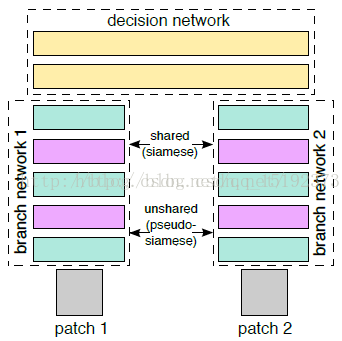## 伪孪生神经网络结构

伪孪生圣经网络结构如下图所示：## 可以解决的问题？

• 孪生网络适用于处理两个输入比较类似的情况
• 伪孪生网络设用于处理啷个输入有一定差别的情况

例如，计算两个句子或者词汇的语义相似度，使用Siamese Network比较合适；验证标题与正文的描述是否一致（标题和正文长度差别很大），或者文字是否描述了一幅图片（一个是图片，一个是文字）就应该使用Pseudo-Siamese Network

## 三胞胎网络（Triplet Network）

论文：《Deep metric learning using Triplet network

三个输入：一个正例+两个负例 或者 两个正例+一个负例

训练的目的是让相同类别间的距离尽可能的小，让不同类别间的距离尽可能的大。

训练效果：Triplet在cifar, mnist的数据集上，效果都是很不错的，超过了siamese network。

## 三胞胎网络结构

输入： x − x^{-} x x 是负样本， x + x^{+} x x 是相似正样本。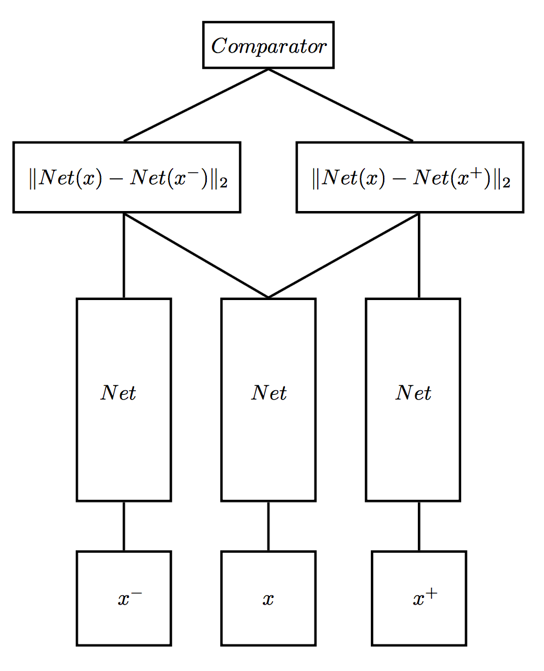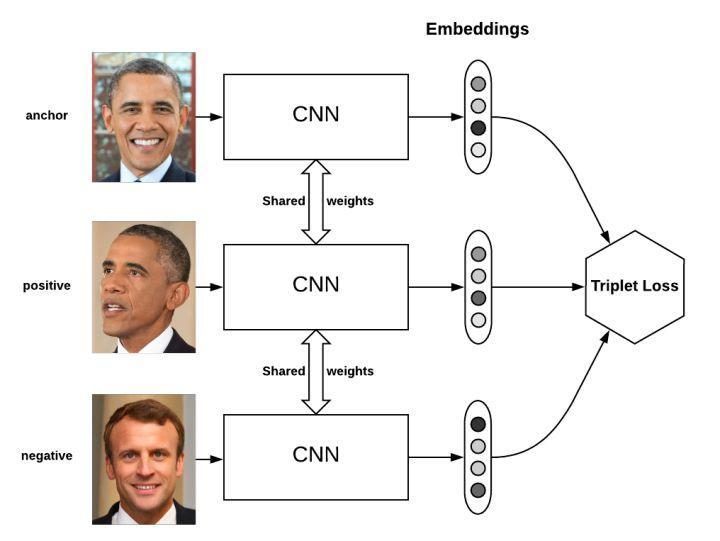## 三胞胎网络的损失函数

损失函数定义如下：
L = max ⁡ ( d ( a , p ) − d ( a , n ) + margin ⁡ , 0 ) \mathcal{L}=\max (d(a, p)-d(a, n)+\operatorname{margin}, 0)

• a a 表示anchor图像
• p p 表示positive图像
• n n 表示negative图像

我们希望 a a p p 的距离应该小于 a a n n 的距离。 m a r g i n margin 是个超参数，它表示 d ( a , p ) d(a,p) d ( a , n ) d(a,n) 之间应该相差多少，例如，假设 m a r g i n = 0 margin=0 ，并且 d ( a , p ) = 0.5 d(a,p)=0.5 ，那么 d ( a , n ) d(a,n) 应该大于等于 0.7 0.7

## Appendix：Andrew NG 三重损失函数

• 代价函数是训练集的所有个体的三重损失的和：
Cost function:  J = ∑ i = 1 n L ( A ( i ) , P ( i ) , N ( i ) ) \text { Cost function: } J=\sum_{i=1}^{n} L\left(A^{(i)}, P^{(i)}, N^{(i)}\right)

• 三重损失函数：
L ( A , P , N ) = max ⁡ ( ∥ f ( A ) − f ( P ) ∥ 2 − ∥ f ( A ) − f ( N ) ∥ 2 + α , 0 ) L(A, P, N)=\max \left(\|f(A)-f(P)\|^{2}-\|f(A)-f(N)\|^{2}+\alpha, 0\right)

• 解释：

这里的最大化处理意味着只要 d ( A , P ) — d ( A , N ) + α d(A, P)—d(A, N)+ α 小于等于 0 0 ，那么 l o s s L ( A , P , N ) loss L(A, P, N) 就会是 0 0 ，但是一旦它大于 0 0 ，那么损失值就是正的，这个函数就会将它最小化成 0 0 或者小于 0 0

这里的问题是，模型可能学习给不同的图片做出相同的编码，这意味着距离会成为 0 0 ，不幸的是，这仍然满足三重损失函数。因为这个原因，我们加入了边际 α α （一个超参数）来避免这种情况的发生。让 d ( A , P ) d(A,P) d ( N , P ) d(N,P) 之间总存在一个差距。

• 为了比较图片 x ( 1 ) x(1) x ( 2 ) x(2) ，我们计算了编码 结果 f ( x 1 ) f(x_{1}) f ( x 2 ) f(x_{2}) 之间的距离。
d ( x ( 1 ) , x ( 2 ) ) = ∥ f ( x ( 1 ) ) − f ( x ( 2 ) ) ∥ 2 2 d\left(x^{(1)}, x^{(2)}\right)=\left\|f\left(x^{(1)}\right)-f\left(x^{(2)}\right)\right\|_{2}^{2}
上式是 x 1 x_{1} x 2 x_{2} 的编码距离。

如果它比某个阈值（一个超参数）小，则意味着两张图片是同一个人，否则，两张图片中不是同一个人。

• 适用于任何两张图片【存在正负样本】

If x ( i ) , x ( j ) x^{(i)}, x^{(j)} are the same person, ∥ f ( x ( i ) ) − f ( x ( j ) ) ∥ 2 \left\|f\left(x^{(i)}\right)-f\left(x^{(j)}\right)\right\|^{2} is small.

If x ( i ) , x ( j ) x^{(i)}, x^{(j)} are different persons, ∥ f ( x ( i ) ) − f ( x ( j ) ) ∥ 2 \left\|f\left(x^{(i)}\right)-f\left(x^{(j)}\right)\right\|^{2} is large.

• 应用：

• Image ranking
• face verification
• metric learning

## 参考资料：

更多相关内容
• Siamese：孪生神经网络在Pytorch当中的实现 目录 实现的内容 该仓库实现了孪生神经网络（Siamese network），该网络常常用于检测输入进来的两张图片的相似性。该仓库所使用的主干特征提取网络（backbone）为VGG16。 ...
• SiamFC孪生神经网络目标跟踪（python代码）
• Siamese：孪生神经网络在Keras当中的实现 目录 实现的内容 该仓库实现了孪生神经网络（Siamese network），该网络常常用于检测输入进来的两张图片的相似性。该仓库所使用的主干特征提取网络（backbone）为VGG16。 所...
• Siamese：孪生神经网络在tf2（tensorflow2）当中的实现 目录 实现的内容 该仓库实现了孪生神经网络（Siamese network），该网络常常用于检测输入进来的两张图片的相似性。该仓库所使用的主干特征提取网络（backbone...Python
• 基于孪生神经网络的主观题自动阅卷评分技术.pdf
• 基于孪生神经网络的跟踪算法是利用离线训练的网络提取目标的特征并进行匹配,从而实现跟踪。在离线训练过程中,网络学到的是相似目标的通用特征,因此当有相似目标干扰时,用这种通用特征表达特定目标将会导致跟踪性能...
• 一、孪生神经网络（Siamese network） 孪生神经网络简单的来说就是权重共享的网络，如下所示： 大家可能会有疑问：共享权值是什么意思？左右两个神经网络的权重一模一样？ 答：是的，在代码实现的时候，甚至...

# 一、孪生神经网络（Siamese network）

## 1.1 网络介绍

孪生神经网络简单的来说就是权重共享的网络，如下所示：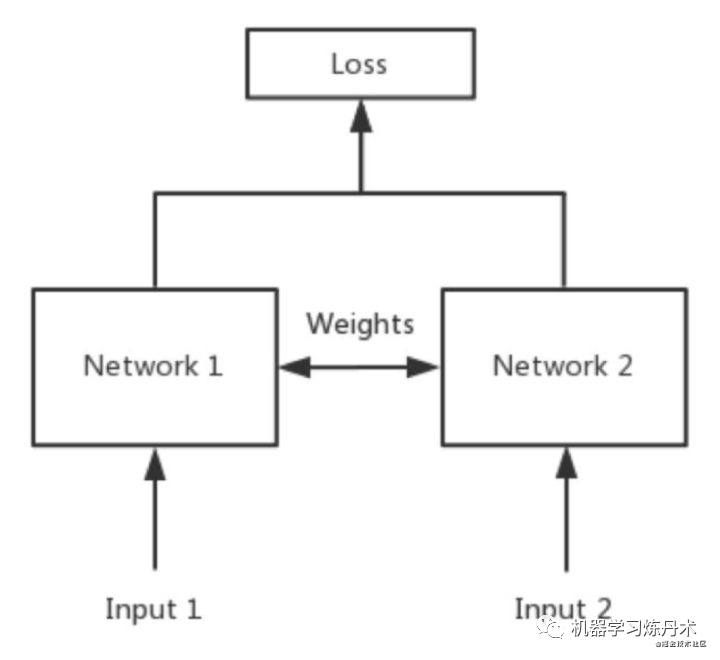从上图我们看到，Network1和Network2共享权重，实际上在操作中这就是一个网络。孪生网络是一种模型，图中的Network可以是CNN，也可是ResNet 等等其他网络。Network1和Network2可以是同一种网络，这时候在实际操作中就相当于是一个网络，同时Network1和Network2也可以是不同的网络，也就是说Network1可以是CNN而同时Network2可以是ResNet，这种时候网络叫做伪孪生神经网络

孪生神经网络和伪孪生神经网络分别适用于什么场景呢？

先上结论：孪生神经网络用于处理两个输入"比较类似"的情况。伪孪生神经网络适用于处理两个输入"有一定差别"的情况。比如，我们要计算两个句子或者词汇的语义相似度，使用siamese network比较适合；如果验证标题与正文的描述是否一致（标题和正文长度差别很大），或者文字是否描述了一幅图片（一个是图片，一个是文字），就应该使用pseudo-siamese network。也就是说，要根据具体的应用，判断应该使用哪一种结构，哪一种Loss。

一般网络是一个input，然后产生一个pred，将这个pred和ground truth进行比较，在返回来修正weigth。而孪生网络需要两个input，得到两个pred，然后产生一个loss。

一般来说，孪生网络是衡量两个输入的关系，也就是两个样本相似还是不相似。

举例：

有这样的一个任务，在NIPS上，在1993年发表了文章《Signature Verification using a ‘Siamese’ Time Delay Neural Network》用于美国支票上的签名验证，检验支票上的签名和银行预留的签名是否一致。当时论文中就已经用卷积网络来做验证了...当时我还没出生。

之后，2010年Hinton在ICML上发表了《Rectified Linear Units Improve Restricted Boltzmann Machines》，用来做人脸验证，效果很好。输入就是两个人脸，输出就是same or different。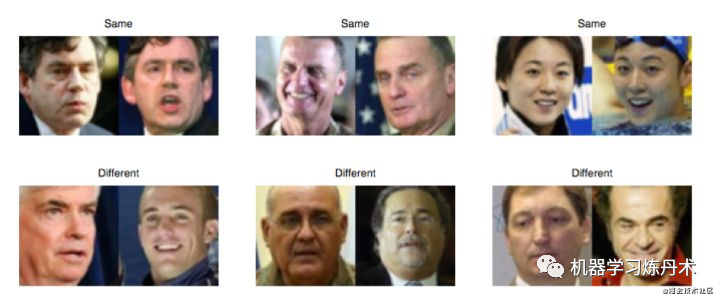还有基于Siamese网络的视觉跟踪算法，比如：Fully-convolutional siamese networks for object tracking

其中，还有人曾经使用三个输入的网络。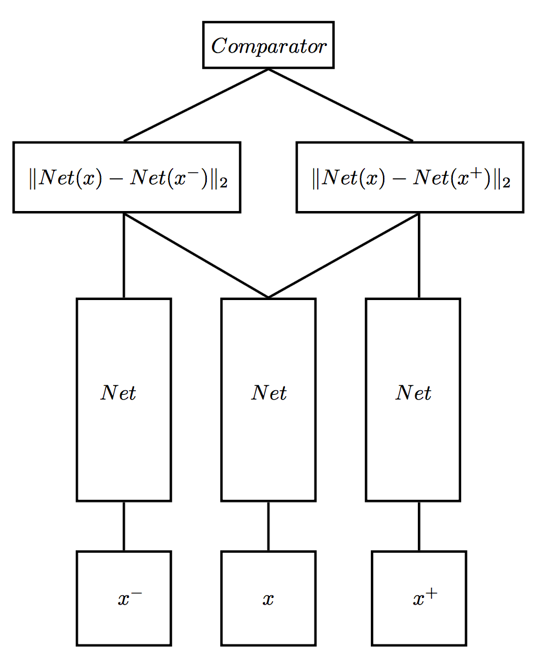这个网络中，属于正样本，就是跟相近的样本 , 而

论文是《Deep metric learning using Triplet network》，输入是三个，一个正例+两个负例，或者一个负例+两个正例，训练的目标是让相同类别间的距离尽可能的小，让不同类别间的距离尽可能的大。

讨论一下孪生神经网络应用场景

• 用于处理类别多（或类别数量不确定）、每一类样本少的分类任务。
• 一般碰到的分类问题都是类别较少，每一类样本较多的情况（如ImageNet的图像分类任务）

## 1.2 损失函数

在孪生神经网络中，一般使用的损失函数为Contrastive Loss.

其定义为：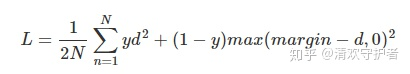对这个公式进行分析：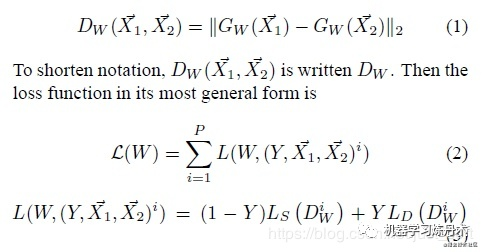网络的两个输入x_1和x_2通过网络G_w后获得两个向量，计算这两个向量之间的欧式距离。然后是关于输入的样本对贴标签，如果输入样本对为正样本对，也就是二者是相似的话，那么Y=0，如果输入的样本对是负样本对，也就是二者区别很大的话，那么Y=1。

则如果是正样本对，则为

如果是负样本,则为

我们训练loss函数的目的是当输出满足要求的话，那么loss函数的值很低。但是如果是负样本的话，二者区别越大，输出的loss函数就越大，这显然不符合我们之前的假设。增加一个margin，当作最大的距离。如果pred1和pred2的距离大于margin，那么就认为这两个样本距离足够大，就当其的损失为0。所以写的方法就是：

在论文中，都设置为0.5

因此上述的Loss函数可以变为N应该是表示输入数据的数量。

## 1.3 代码实现

简单来说，孪生网络就是一次学习两张图片(两张图片是一类或者不是一类)，从中发现它们的相似或者不同，等网络学习完成之后，再给网络输入两张图片即可知道它们是否是一类。

代码实现包括一下几个步骤：

• 1 准备数据
• 2 构建Dataset和可视化
• 3 构建模型
• 4 训练

首先是准备数据：

import pandas as pd
import numpy as np
import matplotlib.pyplot as plt
import torch
import torch.nn as nn
import torch.nn.functional as F
from sklearn.model_selection import train_test_split
device = 'cuda' if torch.cuda.is_available() else 'cpu'
data_train = pd.read_csv('../input/fashion-mnist_train.csv')
data_train.head()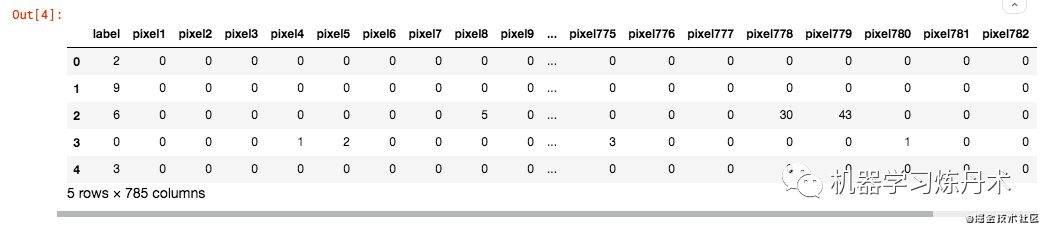这个数据文件是csv格式，第一列是类别，之后的784列其实好似28x28的像素值。

划分训练集和验证集，然后把数据转换成28x28的图片

X_full = data_train.iloc[:,1:]
y_full = data_train.iloc[:,:1]
x_train, x_test, y_train, y_test = train_test_split(X_full, y_full, test_size = 0.05)
x_train = x_train.values.reshape(-1, 28, 28, 1).astype('float32') / 255.
x_test = x_test.values.reshape(-1, 28, 28, 1).astype('float32') / 255.
y_train.label.unique()
>>> array([8, 9, 7, 6, 4, 2, 3, 1, 5, 0])

可以看到这个Fashion MNIST数据集中也是跟MNIST类似，划分了10个不同的类别。

• 0 T-shirt/top
• 1 Trouser
• 2 Pullover
• 3 Dress
• 4 Coat
• 5 Sandal
• 6 Shirt
• 7 Sneaker
• 8 Bag
• 9 Ankle boot

接下来就是数据可视化和数据准备

class mydataset(Dataset):
def __init__(self,x_data,y_data):
self.x_data = x_data
self.y_data = y_data.label.values
def __len__(self):
return len(self.x_data)
def __getitem__(self,idx):
img1 = self.x_data[idx]
y1 = self.y_data[idx]
if np.random.rand() < 0.5:
idx2 = np.random.choice(np.arange(len(self.y_data))[self.y_data==y1],1)
else:
idx2 = np.random.choice(np.arange(len(self.y_data))[self.y_data!=y1],1)
img2 = self.x_data[idx2]
y2 = self.y_data[idx2]
label = 0 if y1==y2 else 1
return img1,img2,label

上面的逻辑就是，给定一个idx，然后我们先判断，这个数据是找两个同类别的图片还是两个不同类别的图片。50%的概率选择两个同类别的图片，然后最后输出的时候，输出这两个图片，然后再输出一个label，这个label为0的时候表示两个图片的类别是相同的，1表示两个图片的类别是不同的。这样就可以进行模型训练和损失函数的计算了。

仔细研究上面的代码，它的返回值两张照片和一个标签。这一步应该是对数据集进行处理，生成图像对，以及图像对的标签，也就是说这一步的操作只是针对于孪生神经网络。

下面一段的代码就是对一个batch的数据进行一个可视化：

for idx,(img1,img2,target) in enumerate(train_dataloader):
fig, axs = plt.subplots(2, img1.shape, figsize = (12, 6))
for idx,(ax1,ax2) in enumerate(axs.T):
ax1.imshow(img1[idx,:,:,0].numpy(),cmap='gray')
ax1.set_title('image A')
ax2.imshow(img2[idx,:,:,0].numpy(),cmap='gray')
ax2.set_title('{}'.format('same' if target[idx]==0 else 'different'))
break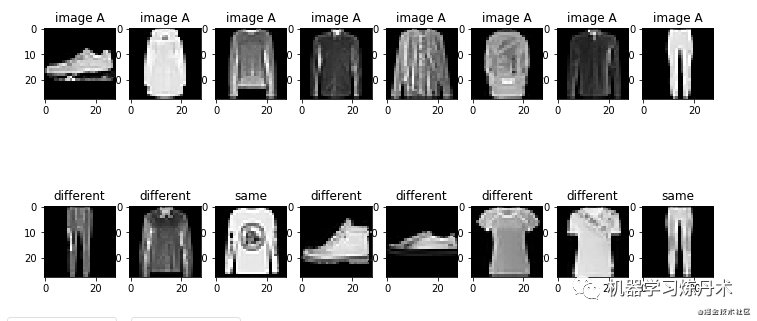接下来就是构建模型

 def __init__(self,z_dimensions=2):
super(siamese,self).__init__()
self.feature_net = nn.Sequential(
nn.ReLU(inplace=True),
nn.BatchNorm2d(4),
nn.ReLU(inplace=True),
nn.BatchNorm2d(4),
nn.MaxPool2d(2),
nn.ReLU(inplace=True),
nn.BatchNorm2d(8),
nn.ReLU(inplace=True),
nn.BatchNorm2d(8),
nn.MaxPool2d(2),
nn.ReLU(inplace=True)
)
self.linear = nn.Linear(49,z_dimensions)
def forward(self,x):
x = self.feature_net(x)
x = x.view(x.shape,-1)
x = self.linear(x)
return x

一个非常简单的卷积网络，输出的向量的维度就是z-dimensions的大小

这个卷积网络5个卷积层。

BatchNorm2d：在卷积神经网络的卷积层之后总会添加BatchNorm2d进行数据的归一化处理，这使得数据在进行Relu之前不会因为数据过大而导致网络性能的不稳定。

MaxPool2d：减小卷积层参数误差造成估计均值的偏移的误差，更多的保留纹理信息

然后就是构造损失函数：

def contrastive_loss(pred1,pred2,target):
MARGIN = 2
euclidean_dis = F.pairwise_distance(pred1,pred2)
target = target.view(-1)
loss = (1-target)*torch.pow(euclidean_dis,2) + target * torch.pow(torch.clamp(MARGIN-euclidean_dis,min=0),2)
return loss

之后就是训练模型

model = siamese(z_dimensions=8).to(device)
optimizor = torch.optim.Adam(model.parameters(),lr=0.001)
for e in range(10):
history = []
img1 = img1.to(device)
img2 = img2.to(device)
target = target.to(device)

pred1 = model(img1)
pred2 = model(img2)
loss = contrastive_loss(pred1,pred2,target)

loss.backward()
optimizor.step()

loss = loss.detach().cpu().numpy()
history.append(loss)
train_loss = np.mean(history)
history = []
img1 = img1.to(device)
img2 = img2.to(device)
target = target.to(device)

pred1 = model(img1)
pred2 = model(img2)
loss = contrastive_loss(pred1,pred2,target)

loss = loss.detach().cpu().numpy()
history.append(loss)
val_loss = np.mean(history)
print(f'train_loss:{train_loss},val_loss:{val_loss}')

这里为了加快训练，我把batch-size增加到了128个，其他的并没有改变：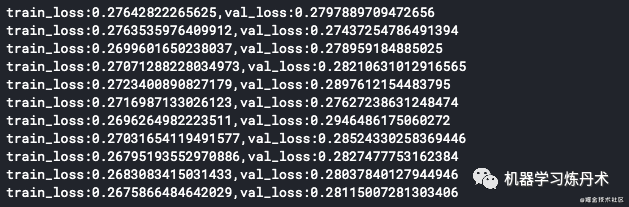接下来就是记得保存模型

torch.save(model.state_dict(),'saimese.pth')

差不多是这个样子，然后看一看验证集的可视化效果，这里使用的是t-sne高位特征可视化的方法，其内核是PCA降维：

from sklearn import manifold
'''X是特征，不包含target;X_tsne是已经降维之后的特征'''
tsne = manifold.TSNE(n_components=2, init='pca', random_state=501)
X_tsne = tsne.fit_transform(X)
print("Org data dimension is {}. \
Embedded data dimension is {}".format(X.shape[-1], X_tsne.shape[-1]))

x_min, x_max = X_tsne.min(0), X_tsne.max(0)
X_norm = (X_tsne - x_min) / (x_max - x_min)  # 归一化
plt.figure(figsize=(8, 8))
for i in range(10):
plt.scatter(X_norm[y==i][:,0],X_norm[y==i][:,1],alpha=0.3,label=f'{i}')
plt.legend()这里的可视化是将高维的特征采用PCA之后降维成像。

展开全文深度学习
• 神经网络学习小记录52——Pytorch 搭建孪生神经网络比较图片相似性学习前言什么是孪生神经网络孪生神经网络的实现思路一、预测部分1、主干网络介绍2、比较网络二、训练部分1、数据集的格式2、Loss计算训练自己的孪生...

# 学习前言

也学习一下Pytorch版本的。# 什么是孪生神经网络

简单来说，孪生神经网络（Siamese network）就是“连体的神经网络”，神经网络的“连体”是通过共享权值来实现的，如下图所示。所谓权值共享就是当神经网络有两个输入的时候，这两个输入使用的神经网络的权值是共享的（可以理解为使用了同一个神经网络）。

很多时候，我们需要去评判两张图片的相似性，比如比较两张人脸的相似性，我们可以很自然的想到去提取这个图片的特征再进行比较，自然而然的，我们又可以想到利用神经网络进行特征提取
如果使用两个神经网络分别对图片进行特征提取，提取到的特征很有可能不在一个域中，此时我们可以考虑使用一个神经网络进行特征提取再进行比较。这个时候我们就可以理解孪生神经网络为什么要进行权值共享了。

孪生神经网络有两个输入（Input1 and Input2），利用神经网络将输入映射到新的空间，形成输入在新的空间中的表示。通过Loss的计算，评价两个输入的相似度。

# 代码下载

https://github.com/bubbliiiing/Siamese-pytorch

# 孪生神经网络的实现思路

## 一、预测部分

### 1、主干网络介绍孪生神经网络的主干特征提取网络的功能是进行特征提取，各种神经网络都可以适用，本文使用的神经网络是VGG16。
关于VGG的介绍大家可以看我的另外一篇博客https://blog.csdn.net/weixin_44791964/article/details/102779878这是一个VGG被用到烂的图，但确实很好的反应了VGG的结构：
1、一张原始图片被resize到指定大小，本文使用105x105。
2、conv1包括两次[3,3]卷积网络，一次2X2最大池化，输出的特征层为64通道。
3、conv2包括两次[3,3]卷积网络，一次2X2最大池化，输出的特征层为128通道。
4、conv3包括三次[3,3]卷积网络，一次2X2最大池化，输出的特征层为256通道。
5、conv4包括三次[3,3]卷积网络，一次2X2最大池化，输出的特征层为512通道。
6、conv5包括三次[3,3]卷积网络，一次2X2最大池化，输出的特征层为512通道。

实现代码为：

import torch
import torch.nn as nn

class VGG(nn.Module):
def __init__(self, features, num_classes=1000):
super(VGG, self).__init__()
self.features = features
self.classifier = nn.Sequential(
nn.Linear(512 * 7 * 7, 4096),
nn.ReLU(True),
nn.Dropout(),
nn.Linear(4096, 4096),
nn.ReLU(True),
nn.Dropout(),
nn.Linear(4096, num_classes),
)
self._initialize_weights()

def forward(self, x):
x = self.features(x)
x = self.avgpool(x)
x = torch.flatten(x, 1)
x = self.classifier(x)
return x

def _initialize_weights(self):
for m in self.modules():
if isinstance(m, nn.Conv2d):
nn.init.kaiming_normal_(m.weight, mode='fan_out', nonlinearity='relu')
if m.bias is not None:
nn.init.constant_(m.bias, 0)
elif isinstance(m, nn.BatchNorm2d):
nn.init.constant_(m.weight, 1)
nn.init.constant_(m.bias, 0)
elif isinstance(m, nn.Linear):
nn.init.normal_(m.weight, 0, 0.01)
nn.init.constant_(m.bias, 0)

def make_layers(cfg, batch_norm=False):
layers = []
in_channels = 1
for v in cfg:
if v == 'M':
layers += [nn.MaxPool2d(kernel_size=2, stride=2)]
else:
conv2d = nn.Conv2d(in_channels, v, kernel_size=3, padding=1)
if batch_norm:
layers += [conv2d, nn.BatchNorm2d(v), nn.ReLU(inplace=True)]
else:
layers += [conv2d, nn.ReLU(inplace=True)]
in_channels = v
return nn.Sequential(*layers)

cfgs = {
'D': [64, 64, 'M', 128, 128, 'M', 256, 256, 256, 'M', 512, 512, 512, 'M', 512, 512, 512, 'M']
}

def VGG16(pretrained, **kwargs):
model = VGG(make_layers(cfgs["D"], batch_norm=False), **kwargs)
if pretrained:
return model


### 2、比较网络在获得主干特征提取网络之后，我们可以获取到一个多维特征，我们可以使用flatten的方式将其平铺到一维上，这个时候我们就可以获得两个输入的一维向量了

将这两个一维向量进行相减，再进行绝对值求和，相当于求取了两个特征向量插值的L1范数。也就相当于求取了两个一维向量的距离。

然后对这个距离再进行两次全连接，第二次全连接到一个神经元上，对这个神经元的结果取sigmoid，使其值在0-1之间，代表两个输入图片的相似程度。

实现代码如下：

import torch
import torch.nn as nn
from nets.vgg import VGG16

def get_img_output_length(width, height):
def get_output_length(input_length):
# input_length += 6
filter_sizes = [2, 2, 2, 2, 2]
padding = [0, 0, 0, 0, 0]
stride = 2
for i in range(5):
input_length = (input_length+2*padding[i]-filter_sizes[i]) // stride + 1
return input_length
return get_output_length(width)*get_output_length(height)

class Siamese(nn.Module):
def __init__(self, input_shape, pretrained=False):
super(Siamese, self).__init__()
self.vgg = VGG16(pretrained)
del self.vgg.avgpool
del self.vgg.classifier

flat_shape = 512 * get_img_output_length(input_shape,input_shape)
self.fully_connect1 = torch.nn.Linear(flat_shape,512)
self.fully_connect2 = torch.nn.Linear(512,1)

def forward(self, x):
x1, x2 = x
x1 = self.vgg.features(x1)
x2 = self.vgg.features(x2)
b, _, _, _ = x1.size()
x1 = x1.view([b,-1])
x2 = x2.view([b,-1])
x = torch.abs(x1-x2)
x = self.fully_connect1(x)
x = self.fully_connect2(x)
return x


## 二、训练部分

### 1、数据集的格式

本文所使用的数据集为Omniglot数据集。
其包含来自 50不同字母（语言）的1623 个不同手写字符。每一个字符都是由 20个不同的人通过亚马逊的 Mechanical Turk 在线绘制的。

相当于每一个字符有20张图片，然后存在1623个不同的手写字符，我们需要利用神经网络进行学习，去区分这1623个不同的手写字符，比较输入进来的字符的相似性。

本博客中数据存放格式有三级：

- image_background
- Alphabet_of_the_Magi
- character01
- 0709_01.png
- 0709_02.png
- ……
- character02
- character03
- ……
- Anglo-Saxon_Futhorc
- ……


最后一级的文件夹用于分辨不同的字体，同一个文件夹里面的图片属于同一文字。在不同文件夹里面存放的图片属于不同文字。上两个图为.\images_background\Alphabet_of_the_Magi\character01里的两幅图。它们两个属于同一个字。上一个图为.\images_background\Alphabet_of_the_Magi\character02里的一幅图。它和上面另两幅图不属于同一个字。

### 2、Loss计算

对于孪生神经网络而言，其具有两个输入。

当两个输入指向同一个类型的图片时，此时标签为1。

当两个输入指向不同类型的图片时，此时标签为0。

然后将网络的输出结果和真实标签进行交叉熵运算，就可以作为最终的loss了。

本文所使用的Loss为binary_crossentropy。

当我们输入如下两个字体的时候，我们希望网络的输出为1。我们会将预测结果和1求交叉熵。

当我们输入如下两个字体的时候，我们希望网络的输出为0。我们会将预测结果和0求交叉熵。

# 训练自己的孪生神经网络

## 1、训练本文所使用的Omniglot例子下载数据集，放在根目录下的dataset文件夹下。运行train.py开始训练。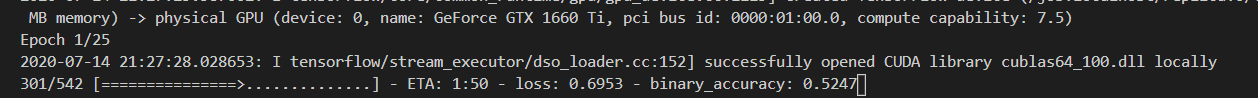## 2、训练自己相似性比较的模型

如果大家想要训练自己的数据集，可以将数据集按照如下格式进行摆放。每一个chapter里面放同类型的图片。
之后将train.py当中的train_own_data设置成True，即可开始训练。展开全文图片相似性 神经网络 深度学习
• 神经网络学习小记录51——Keras 搭建孪生神经网络比较图片相似性学习前言什么是孪生神经网络孪生神经网络的实现思路一、预测部分1、主干网络介绍 学习前言 最近学习了一下如何比较两张图片的相似性，用到了孪生神经...

# 学习前言

最近学习了一下如何比较两张图片的相似性，用到了孪生神经网络，一起来学习一下。# 什么是孪生神经网络

简单来说，孪生神经网络（Siamese network）就是“连体的神经网络”，神经网络的“连体”是通过共享权值来实现的，如下图所示。所谓权值共享就是当神经网络有两个输入的时候，这两个输入使用的神经网络的权值是共享的（可以理解为使用了同一个神经网络）。

很多时候，我们需要去评判两张图片的相似性，比如比较两张人脸的相似性，我们可以很自然的想到去提取这个图片的特征再进行比较，自然而然的，我们又可以想到利用神经网络进行特征提取
如果使用两个神经网络分别对图片进行特征提取，提取到的特征很有可能不在一个域中，此时我们可以考虑使用一个神经网络进行特征提取再进行比较。这个时候我们就可以理解孪生神经网络为什么要进行权值共享了。

孪生神经网络有两个输入（Input1 and Input2），利用神经网络将输入映射到新的空间，形成输入在新的空间中的表示。通过Loss的计算，评价两个输入的相似度。

# 代码下载

https://github.com/bubbliiiing/Siamese-keras

# 孪生神经网络的实现思路

## 一、预测部分

### 1、主干网络介绍孪生神经网络的主干特征提取网络的功能是进行特征提取，各种神经网络都可以适用，本文使用的神经网络是VGG16。
关于VGG的介绍大家可以看我的另外一篇博客https://blog.csdn.net/weixin_44791964/article/details/102779878这是一个VGG被用到烂的图，但确实很好的反应了VGG的结构：
1、一张原始图片被resize到指定大小，本文使用105x105。
2、conv1包括两次[3,3]卷积网络，一次2X2最大池化，输出的特征层为64通道。
3、conv2包括两次[3,3]卷积网络，一次2X2最大池化，输出的特征层为128通道。
4、conv3包括三次[3,3]卷积网络，一次2X2最大池化，输出的特征层为256通道。
5、conv4包括三次[3,3]卷积网络，一次2X2最大池化，输出的特征层为512通道。
6、conv5包括三次[3,3]卷积网络，一次2X2最大池化，输出的特征层为512通道。

实现代码为：

import keras
from keras.layers import Input,Dense,Conv2D
from keras.layers import MaxPooling2D,Flatten
from keras.models import Model
import os
import numpy as np
from PIL import Image
from keras.optimizers import SGD

class VGG16:
def __init__(self):
self.block1_conv1 = Conv2D(64,(3,3),activation = 'relu',padding = 'same',name = 'block1_conv1')
self.block1_conv2 = Conv2D(64,(3,3),activation = 'relu',padding = 'same', name = 'block1_conv2')
self.block1_pool = MaxPooling2D((2,2), strides = (2,2), name = 'block1_pool')

self.block2_conv1 = Conv2D(128,(3,3),activation = 'relu',padding = 'same',name = 'block2_conv1')
self.block2_conv2 = Conv2D(128,(3,3),activation = 'relu',padding = 'same',name = 'block2_conv2')
self.block2_pool = MaxPooling2D((2,2),strides = (2,2),name = 'block2_pool')

self.block3_conv1 = Conv2D(256,(3,3),activation = 'relu',padding = 'same',name = 'block3_conv1')
self.block3_conv2 = Conv2D(256,(3,3),activation = 'relu',padding = 'same',name = 'block3_conv2')
self.block3_conv3 = Conv2D(256,(3,3),activation = 'relu',padding = 'same',name = 'block3_conv3')
self.block3_pool = MaxPooling2D((2,2),strides = (2,2),name = 'block3_pool')

self.block4_conv1 = Conv2D(512,(3,3),activation = 'relu',padding = 'same', name = 'block4_conv1')
self.block4_conv2 = Conv2D(512,(3,3),activation = 'relu',padding = 'same', name = 'block4_conv2')
self.block4_conv3 = Conv2D(512,(3,3),activation = 'relu',padding = 'same', name = 'block4_conv3')
self.block4_pool = MaxPooling2D((2,2),strides = (2,2),name = 'block4_pool')

# 第五个卷积部分
self.block5_conv1 = Conv2D(512,(3,3),activation = 'relu',padding = 'same', name = 'block5_conv1')
self.block5_conv2 = Conv2D(512,(3,3),activation = 'relu',padding = 'same', name = 'block5_conv2')
self.block5_conv3 = Conv2D(512,(3,3),activation = 'relu',padding = 'same', name = 'block5_conv3')
self.block5_pool = MaxPooling2D((2,2),strides = (2,2),name = 'block5_pool')

self.flatten = Flatten(name = 'flatten')

def call(self, inputs):
x = inputs
x = self.block1_conv1(x)
x = self.block1_conv2(x)
x = self.block1_pool(x)

x = self.block2_conv1(x)
x = self.block2_conv2(x)
x = self.block2_pool(x)

x = self.block3_conv1(x)
x = self.block3_conv2(x)
x = self.block3_conv3(x)
x = self.block3_pool(x)

x = self.block4_conv1(x)
x = self.block4_conv2(x)
x = self.block4_conv3(x)
x = self.block4_pool(x)

x = self.block5_conv1(x)
x = self.block5_conv2(x)
x = self.block5_conv3(x)
x = self.block5_pool(x)

outputs = self.flatten(x)
return outputs


### 2、比较网络在获得主干特征提取网络之后，我们可以获取到一个多维特征，我们可以使用flatten的方式将其平铺到一维上，这个时候我们就可以获得两个输入的一维向量了

将这两个一维向量进行相减，再进行绝对值求和，相当于求取了两个特征向量插值的L1范数。也就相当于求取了两个一维向量的距离。

然后对这个距离再进行两次全连接，第二次全连接到一个神经元上，对这个神经元的结果取sigmoid，使其值在0-1之间，代表两个输入图片的相似程度。

实现代码如下：

import keras
from keras.layers import Input,Dense,Conv2D
from keras.layers import MaxPooling2D,Flatten,Lambda
from keras.models import Model
import keras.backend as K
import os
import numpy as np
from PIL import Image
from keras.optimizers import SGD
from nets.vgg import VGG16

def siamese(input_shape):
vgg_model = VGG16()

input_image_1 = Input(shape=input_shape)
input_image_2 = Input(shape=input_shape)

encoded_image_1 = vgg_model.call(input_image_1)
encoded_image_2 = vgg_model.call(input_image_2)

l1_distance_layer = Lambda(
lambda tensors: K.abs(tensors - tensors))
l1_distance = l1_distance_layer([encoded_image_1, encoded_image_2])

out = Dense(512,activation='relu')(l1_distance)
out = Dense(1,activation='sigmoid')(out)

model = Model([input_image_1,input_image_2],out)
return model


## 二、训练部分

### 1、数据集的格式

本文所使用的数据集为Omniglot数据集。
其包含来自 50不同字母（语言）的1623 个不同手写字符。每一个字符都是由 20个不同的人通过亚马逊的 Mechanical Turk 在线绘制的。

相当于每一个字符有20张图片，然后存在1623个不同的手写字符，我们需要利用神经网络进行学习，去区分这1623个不同的手写字符，比较输入进来的字符的相似性。

本博客中数据存放格式有三级：

- image_background
- Alphabet_of_the_Magi
- character01
- 0709_01.png
- 0709_02.png
- ……
- character02
- character03
- ……
- Anglo-Saxon_Futhorc
- ……


最后一级的文件夹用于分辨不同的字体，同一个文件夹里面的图片属于同一文字。在不同文件夹里面存放的图片属于不同文字。上两个图为.\images_background\Alphabet_of_the_Magi\character01里的两幅图。它们两个属于同一个字。上一个图为.\images_background\Alphabet_of_the_Magi\character02里的一幅图。它和上面另两幅图不属于同一个字。

### 2、Loss计算

对于孪生神经网络而言，其具有两个输入。

当两个输入指向同一个类型的图片时，此时标签为1。

当两个输入指向不同类型的图片时，此时标签为0。

然后将网络的输出结果和真实标签进行交叉熵运算，就可以作为最终的loss了。

本文所使用的Loss为binary_crossentropy。

当我们输入如下两个字体的时候，我们希望网络的输出为1。我们会将预测结果和1求交叉熵。

当我们输入如下两个字体的时候，我们希望网络的输出为0。我们会将预测结果和0求交叉熵。

# 训练自己的孪生神经网络

## 1、训练本文所使用的Omniglot例子下载数据集，放在根目录下的dataset文件夹下。运行train.py开始训练。## 2、训练自己相似性比较的模型

如果大家想要训练自己的数据集，可以将数据集按照如下格式进行摆放。每一个chapter里面放同类型的图片。
之后将train.py当中的train_own_data设置成True，即可开始训练。展开全文Siamese keras 神经网络 深度学习
• 针对全卷积孪生网络目标跟踪算法(Siamfc)在严重遮挡、旋转、光照变化、尺度变化等情况下容易出现跟踪失败的问题,提出了一种融合扰动感知模型的孪生神经网络目标跟踪算法。将孪生神经网络提取到的低层结构特征与高层...
• 孪生神经网络 定义 孪生神经网络（Siamese network）就是“连体的神经网络”，神经网络的“连体”是通过共享权值来实现的。 当我们想要提取同一属性的特征的时候，如果使用两个神经网络分别对图片进行特征提取，...
• Siamese在英语中是“孪生”、“连体”的意思，这是为什么呢？十九世纪泰国出生了一对连体婴儿，当时的医学技术无法使两人分离出来，于是两人顽强地生活了一生，1829年被英国商人发现，进入马戏团，在全世界各地表演...
• 神经网络学习小记录53——TF2搭建孪生神经网络比较图片相似性（tensorflow2）学习前言什么是孪生神经网络孪生神经网络的实现思路一、预测部分1、主干网络介绍2、比较网络二、训练部分1、数据集的格式2、Loss计算训练...Siamese 深度学习 卷积
• 孪生神经网络（SNN）是一种神经网络，其中包含相同模型的多个实例，并共享相同的体系结构和权重。当需要使用有限的数据进行学习并且我们没有完整的数据集（例如零/一枪学习任务）时，这种架构就显示了其优势。 传统...神经网络 深度学习 python 人工智能
• 本文是论文《ChangeNet: A Deep Learning Architecture ...本文提出了一个基于深度学习的变化检测网络ChangeNet。 一、相关工作 给定测试图像ItestI_{test}Itest​和参考图像IrefI_{ref}Iref​，它们的大小都为w×h×dChangeNet
• ---------搭建网络框架------------ class Siamese(nn.Module): def __init__(self): super(Siamese, self).__init__() self.conv1 = nn.Sequential( nn.Conv2d( in_channels=3,#通道数目，刚输入的图片是彩色的三...pytorch 深度学习
• 孪生神经网路SiameseNetwork介绍及基于Pytorch的实现
• 孪生神经网络有两个输入（Input1 and Input2）,将两个输入feed进入两个神经网络（Network1 and Network2），这两个神经网络分别将输入映射到新的空间，形成输入在新的空间中的表示。通过Loss的计算，评价两个输入的...机器学习
• 孪生神经网络 02 孪生神经网络的用途是什么？ 简单来说，衡量两个输入的相似程度。孪生神经网络有两个输入（Input1 and Input2）,将两个输入feed进入两个神经网络（Network1 and Network2），这两个神经网络分别...
• 根据网络的结构，现在变化检测的模型主要有三类，一是基于孪生神经网络的，二是基于伪孪生神经网络的，三是基于两通道的，孪生神经网络的两个分支是共享参数的，而伪孪生神经网络的两个分支是不共享参数的，两通道的...
• 这种分类问题可以使用神经网络或者SVM解决，只要事先知道了所有的类。 第二类，分类数量较多（或者说无法确认具体数量），每一类的数据量较少，比如人脸识别、人脸验证任务。 2. 文章创新点 解决以上两个问题，本文...siamese 人脸识别 计算机视觉 深度学习
• 孪生网络发展总结：https://blog.csdn.net/haoji007/article/details/88387420 实验: 1.不同深度直接的对比 2.融合实验，为了证明假说：CF在训练中就融入会比跟踪时融入好 3.自适应实验，给了一个不变的CF层验证...
• 孪生神经网络实现目标跟踪，可自行训练，跟踪率在15fps以下
• 基于形状描述符和孪生神经网络的纹理分割算法.pdf
• 在往期的神经网络中，我们训练样本的时候需要成千上万的样本数据，在对这些数据进行收集和打标签的时候，往往需要付出比较多的代价。比如我们需要采集某个型号的设备开启时一段时间内的信号，那么我们需要对该种型号...机器学习 深度学习 人工智能 tensorflow
• 1.孪生神经网络(Siamese Networks) 1.1 基本概念 同义与歧义： 同义：两个完全不同的句子可能有相同的意思 歧义：两个基本相同的句子可能有完全不同的意思 定义：由左右两个神经网络构成，两个网络共享......